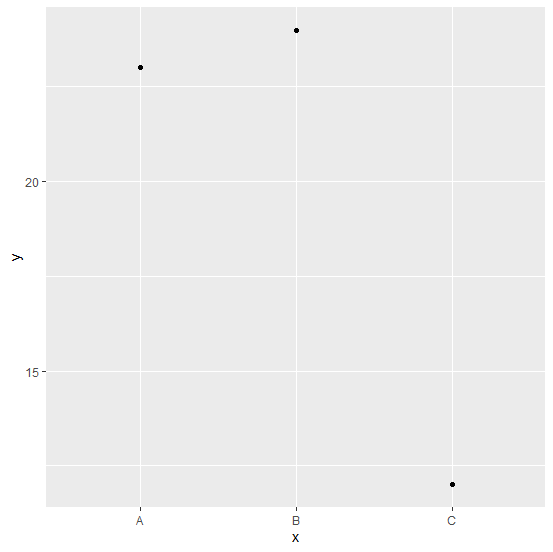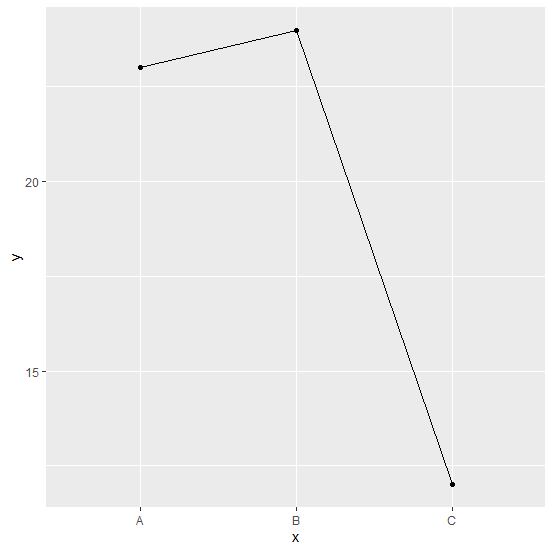# How to join the points of a point chart if X-axis values are categorical in R using ggplot2?

A point chart is usually drawn to see the relationship between two continuous variables and it is also called scatterplot but if the independent variable is categorical then we simply call it a point chart. Often, we want to join or connect the points of a point chart to visually represent the variation of categories of the independent variable and make it a line chart. This can be done by setting stat_summary argument geom to line and setting group = 1 in aes.

## Example

Consider the below data frame −

Live Demo

Class<-c("A","B","C")
Frequency<-c(23,24,12)
df<-data.frame(Class,Frequency)
df

## Output

 Class Frequency
1 A 23
2 B 24
3 C 12
library(ggplot2)

Creating a simple point chart −

ggplot(df,aes(x,y,group=1))+geom_point()

## OutputCreating the plot with connected points −

ggplot(df,aes(x,y,group=1))+geom_point()+stat_summary(fun.y=sum,geom="line")

## Output